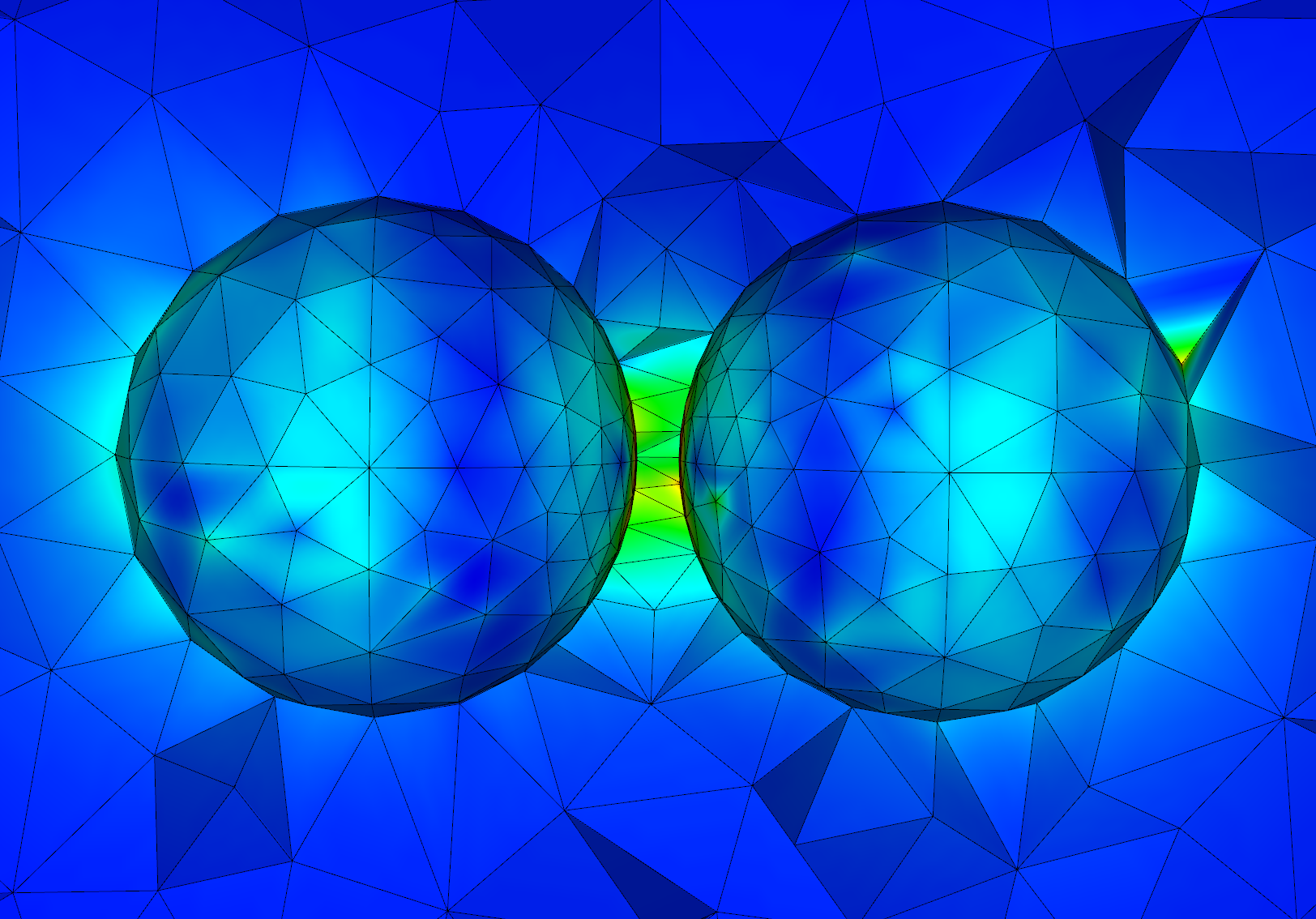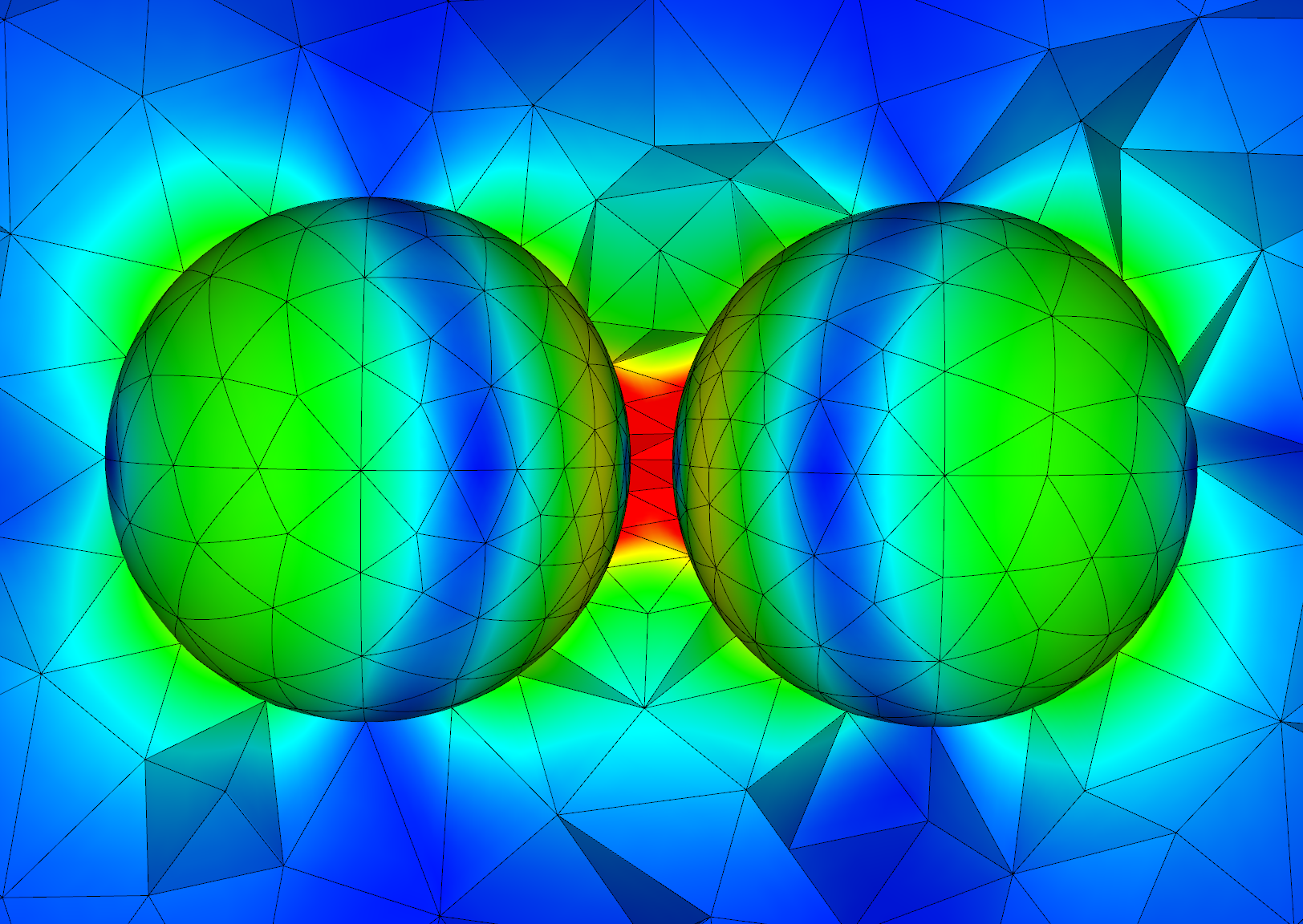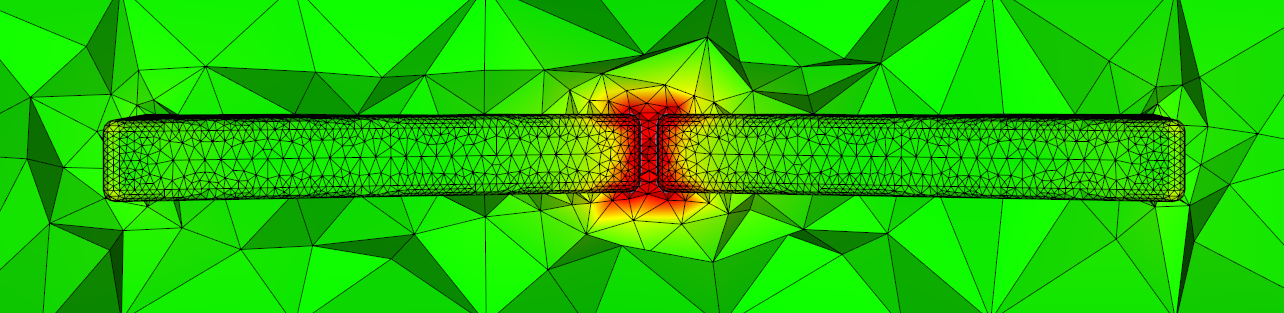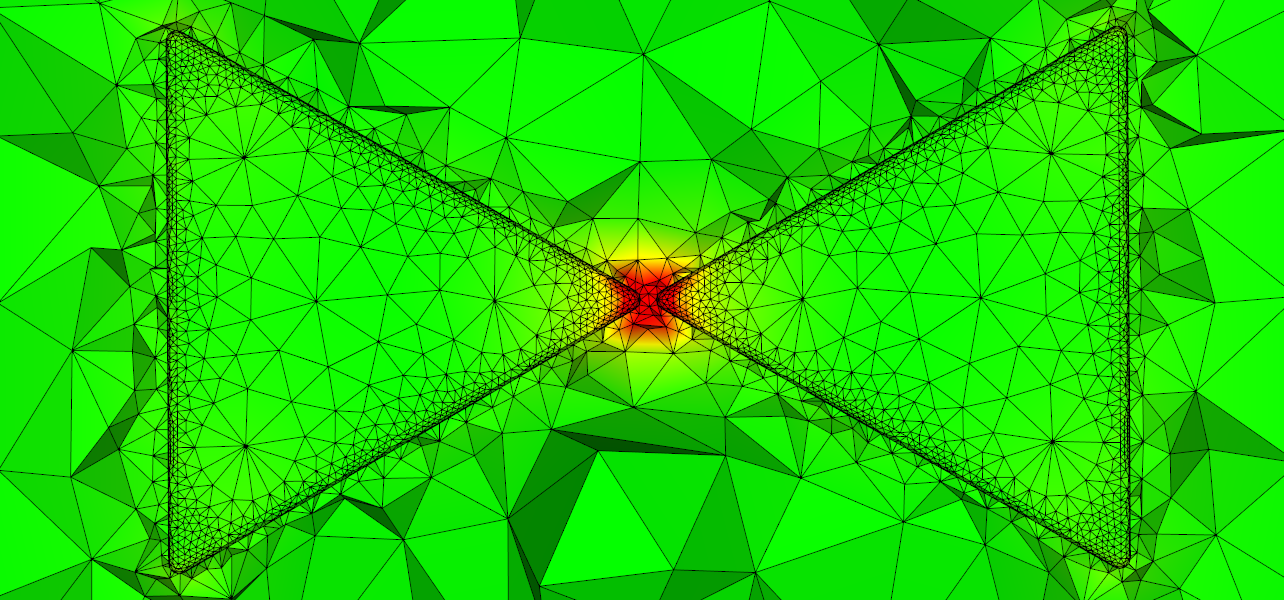EN FR
• Legal notice
• Accessibility - non conforme
##### NACHOS - 2015

Application Domains
New Software and Platforms
Partnerships and Cooperations
Bibliography

## Section: New Results

### Electromagnetic wave propagation

#### Numerical study of the non-linear Maxwell equations for Kerr media

Participants : Loula Fezoui, Stéphane Lanteri.

The system of Maxwell equations describes the evolution of the interaction of an electromagnetic field with a propagation medium. The different properties of the medium, such as isotropy, homogeneity, linearity, among others, are introduced through constitutive laws linking fields and inductions. In the present study, we focus on non-linear effects and address non-linear Kerr materials specifically. In this model, any dielectric may become non-linear provided the electric field in the material is strong enough. As a first setp, we considered the one-dimensional case and study the numerical solution of the non-linear Maxwell equations thanks to DG methods. In particular, we make use of an upwind scheme and limitation techniques because they have a proven ability to capture shocks and other kinds of singularities in the fluid dynamics framework. The numerical results obtained in this preliminary study gave us confidence towards extending them to higher spatial dimensions. As a matter of fact, we recently started to work on the three-dimensional case and have initiated the development of a parallel simulation software based on our past contributions on DGTD methods for the case of linear propagation media.

#### High order geometry conforming DGTD method for nanophotonics

Participants : Stéphane Lanteri, Claire Scheid, Jonathan Viquerat.

Usually, unstructured mesh based methods rely on tessellations composed of straight-edged elements mapped linearly from a reference element, on domains which physical boundaries are indifferently straight or curved. Such meshes represent a serious hindrance for high order finite element (FE) methods since they limit the accuracy to second order in the spatial discretization. Thus, exploiting an enhanced representation of physical geometries is in agreement with the natural procedure of high order FE methods, such as the DG method. There are several ways to account for curved geometries. One could choose to incorporate the knowledge coming from CAD in the method to design the geometry and the approximation. These methods are called isogeometric, and have received a lot of attention recently. This naturally implies to have access to CAD models of the geometry. On the other hand, isoparametric usually rely on a polynomial approximation of both the boundary and the solution. This can be added fairly easily on top of existing implementations. In the present study we focus on the latter type of method, since our goal is first to envisage the benefit of curvilinear meshes for light/matter interaction with nanoscale structures.

Figure 4. Near-field visualization of the amplitude of the electric field Fourier transformed for a gold nanosphere dimer. Surface-to-surface distance is set to 4 nm. Calculations are based on a DGTD-${ℙ}_{4}$ method.Mesh with affine elements Mesh with curvilinear elements

#### Local approximation order DGTD method for nanophotonics

Participants : Stéphane Lanteri, Jonathan Viquerat.

High order DGTD methods for the numerical modeling of light/matter interactions on the nanoscale often assume a uniform distribution of the polynomial order to the cells of the underlying mesh. However, in the case of a mesh showing large variations in cell size, the time step imposed by the smallest cells can be a serious hindrance when trying to exploit high approximation orders. Indeed, a potentially large part of the CPU time is spent in the update of the physical field inside small cells where high polynomial orders might not be essential, while they are necessary in the larger cells. In this study, we consider the possibility of using a non-uniform distribution of the polynomial order in the framework of a global time step DGDT method. By imposing low orders in small cells and high orders in large cells, it is possible to significantly alleviate both the global number of degrees of freedom and the time step restriction with a minimal impact on the method accuracy. Strategies exploiting locally adaptive (LA) formulations usually combine both $h$- and $p$-adaptivity (where $h$ denotes the discretization parameter in space and $p$ the degree of the interpolation of the field components) in order to concentrate the computational effort in the areas of high field variations. Here, the adopted point of view is quite different: starting from a given mesh and a uniform distribution of the polynomial order $k$, the LA strategy exploits all the polynomial orders $p$ with $p\le k$ to obtain a solution of similar accuracy with a reduced computational cost.

Figure 5. Near-field plasmonic interaction with a bowtie nanoantenns: contour line the amplitude of the discrete Fourier transform of the electric field.#### Numerical treatment of non-local dispersion for nanoplasmonics

Participants : Stéphane Lanteri, Claire Scheid, Colin Vo Cong Tri.

When metallic nanostructures have sub-wavelength sizes and the illuminating frequencies are in the regime of metal's plasma frequency, electron interaction with the exciting fields have to be taken into account. Due to these interactions, plasmonic surface waves can be excited and cause extreme local field enhancements (surface plasmon polariton electromagnetic waves). Exploiting such field enhancements in applications of interest requires a detailed knowledge about the occurring fields which can generally not be obtained analytically. For the numerical modeling of light/matter interaction on the nanoscale, the choice of an appropriate model is a crucial point. Approaches that are adopted in a first instance are based on local (no interaction between electrons) dispersion models e.g. Drude or Drude-Lorentz. From the mathematical point of view, these models lead to an additional ordinary differential equation in time that is coupled to Maxwell's equations. When it comes to very small structures in a regime of 2 nm to 25 nm, non-local effects due to electron collisions have to be taken into account. Non-locality leads to additional, in general non-linear, partial differential equations and is significantly more difficult to treat, though. In this work, we study a DGTD method able to solve the system of Maxwell equations coupled to a linearized non-local dispersion model relevant to nanoplasmonics. While the method is presented in the general 3d case, in this preliminary stdudy, numerical results are given for 2d simulation settings.

#### Corner effects in nanoplasmonics

Participants : Camille Carvalho [ENSTA, POEMS project-team] , Patrick Ciarlet [ENSTA, POEMS project-team] , Claire Scheid.

The starting point of this ongoing work is the theoretical and numerical study of nanoplasmonic structures with corners. This is the central subject of the PhD thesis of Camille Carvalho. In the latter, the focus is made on a lossles Drude dispersion model with a frequency domain approach. Several well posedness problems arise due to the presence of corners and are addressed in the PhD thesis. A time domain approach in this context can also be investigated with the techniques developed in our project-team. Even if both approaches (time domain and frequency domain) represent similar physical phenomena, problems that arise are different and they appear as complementary; it is thus worth bridging the gap between the two frameworks. We propose to perform a thorough comparison in the case of structures with corners. Several extensions to other models are also envisaged, especially concerning the non local dispersion model.

#### DGTD method for nanoplasmonics based on generalized dispersion model

Participants : Stéphane Lanteri, Claire Scheid, Jonathan Viquerat.

In this work, we are concerned with the numerical modelling of the propagation of electromagnetic waves in dispersive materials for nanophotonics applications. We focus on a generalized model that allows for the description of a wide range of dispersive media. The underlying differential equations are recast into a generic form and we establish an existence and uniqueness result. We then turn to the numerical treatment and propose an appropriate DGTD framework. We obtain the semi-discrete convergence and prove the stability (and to a large extent, the convergence) in the fully discrete case when time integration is achieved with a 4 steps low storage Runge-Kutta scheme, via a technique relying on energy principles. Finally, we validate our results through the numerical simulation of two nanophotonics test cases.

#### Travelling waves for the non-linear Schrödinger equation in 2d

Participants : David Chiron [J.A. Dieudonné Laboratory, University of Nice-Sophia Antipolis] , Claire Scheid.

We are interested in the numerical study of the two-dimensional travelling waves of the non-linear Schrödinger equation for a general non-linearity and with nonzero condition at infinity that can appear in optics. The equation has a variational structure that we propose to exploit to design a numerical method. We characterize the saddle points of the action as minimizers of another functional, allowing us to use a gradient flow. Combining this approach with a continuation method in the speed of the wave, we obtain the numerical solution for the full range of velocities. We plot the energy-momentum diagrams for different type of non-linearities. Through various examples, we show that even though the non-linearity has the same behaviour as the well-known Gross-Pitaevskii (GP) non-linearity, the qualitative properties of the travelling waves may be extremely different. For instance, we observe cusps, a modified Kadomtsev-Petviashvili I (KP-I) asymptotic in the transonic limit (as the speed of the wave approaches the speed of sound), various multiplicity results.

#### Multiscale DG methods for the time-domain Maxwell equations

Participants : Stéphane Lanteri, Raphaël Léger, Diego Paredes Concha [Instituto de Matemáticas, Universidad Católica de Valparaiso, Chile] , Claire Scheid, Frédéric Valentin [LNCC, Petropolis, Brazil] .

Although the DGTD method has already been successfully applied to complex electromagnetic wave propagation problems, its accuracy may seriously deteriorate on coarse meshes when the solution presents multiscale or high contrast features. In other physical contexts, such an issue has led to the concept of multiscale basis functions as a way to overcome such a drawback and allow numerical methods to be accurate on coarse meshes. The present work, which has been initiated in the context of the visit of Frédéric Valentin in the team, is concerned with the study of a particular family of multiscale methods, named Multiscale Hybrid-Mixed (MHM) methods. Initially proposed for fluid flow problems, MHM methods are a consequence of a hybridization procedure which caracterize the unknowns as a direct sum of a coarse (global) solution and the solutions to (local) problems with Neumann boundary conditions driven by the purposely introduced hybrid (dual) variable. As a result, the MHM method becomes a strategy that naturally incorporates multiple scales while providing solutions with high order accuracy for the primal and dual variables. The completely independent local problems are embedded in the upscaling procedure, and computational approximations may be naturally obtained in a parallel computing environment. In this study, a family of MHM methods is proposed for the solution of the time-domain Maxwell equations where the local problems are discretized either with a continuous FE method or a DG method (that can be viewed as a multiscale DGTD method). Preliminary results have been obtained in the 2d case for models problems.

#### HDG methods for the time-domain Maxwell equations

Participants : Alexandra Christophe-Argenvillier, Stéphane Descombes, Stéphane Lanteri.

This study is concerned with the development of accurate and efficient solution strategies for the system of 3d time-domain Maxwell equations coupled to local dispersion models (e.g. Debye, Drude or Drude-Lorentz models) in the presence of locally refined meshes. Such meshes impose a constraint on the allowable time step for explicit time integration schemes that can be very restrictive for the simulation of 3d problems. We consider here the possibility of using an unconditionally stable implicit time or a locally implicit time integration scheme combined to a HDG discretization method. In doing so, we extend our former study which was dealing with the 2d time-domain Maxwell equations for non-dispersive media.

#### HDG methods for the frequency-domain Maxwell equations

Participants : Thomas Frachon, Stéphane Lanteri, Liang Li [UESTC, Chengdu, China] , Ludovic Moya, Ronan Perrussel [Laplace Laboratory, Toulouse] .

In the context of the ANR TECSER project, we continue our efforts towards the development of scalable high order HDG methods for the solution of the system of 3d frequency-domain Maxwell equations. We aim at fully exploiting the flexibiity of the HDG discretization framework with regards to the adaptation of the interpolation order ($p$-adaptivity) and the mesh ($h$-adaptivity). In particular, we study the formulation of HDG methods on a locally refined non-conforming tetrahedral mesh and on a non-confoming hybrid cubic/tetrahedral mesh. We also investigate the coupling between the HDG formulation and a BEM (Boundary Element Method) discretization of an integral representation of the electromagnetic field in the case of propagation problems theoretically defined in unbounded domains.